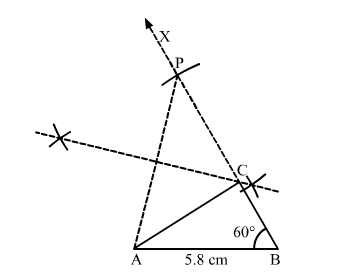# Construct a ∆ABC in which AB = 5.8 cm, ∠B = 60° and BC + CA = 8.4 cm.

Question:

Construct a ∆ABC in which AB = 5.8 cm, ∠= 60° and BC CA = 8.4 cm. Justify your construction.

Solution:Steps of construction:
1. Draw a line segment AB = 5.8 cm.

2. Construct $\angle A B X=60^{\circ}$.

3. Set off BP = 8.4 cm.
4. Join PA.
5. Draw the right bisector of PA, meeting BP at C.
6. Join AC.

Thus, $\triangle A B C$ is the required triangle.

Justification:

In $\triangle \mathrm{APC}$,

∠CAP = ∠CPA                               (By construction)
⇒ CP = AC                  (Sides opposite to equal angles are equal)
Now
BC = PB
− PC = PB − AC
⇒ BC + AC = PB